# Advanced Geometry : How to find the length of an edge

## Example Questions

2 Next →

### Example Question #11 : Tetrahedrons

In order for the height of a regular tetrahedron to be one, what should the lengths of the sides be?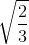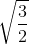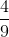Explanation:

The formula for the height of a regular tetrahedron is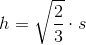, where s is the length of the sides.

In this case we want h to be 1, so we need something that multiplies to 1 with.

We know that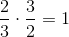, so then we know that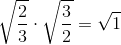, which equals 1.

Therefore s should be.

### Example Question #12 : Tetrahedrons

The volume of a regular tetrahedron is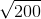. Find the length of one side.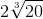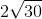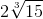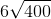Explanation:

The formula for the volume of a regular tetrahedron is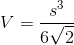.

In this case we know that the volume, V, is, so we can plug that in to solve for s, the length of each edge: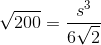[multiply both sides by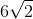]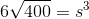[evaluate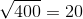and multiply]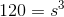[take the cube root of each side]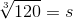We can simplify this by factoring 120 as the product of 8 times 15. Since the cube root of 8 is 2, we get:.

### Example Question #13 : Tetrahedrons

A regular tetrahedron has surface area 1,000. Which of the following comes closest to the length of one edge?Explanation:

A regular tetrahedron has six congruent edges and, as its faces, four congruent equilateral triangles. If we letbe the length of one edge, each face has as its area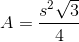;

the total surface area of the tetrahedron is therefore four times this, or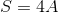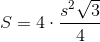Set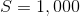and solve for: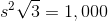Divide by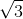: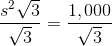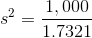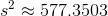Take the square root of both sides: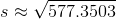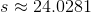Of the given choices, 20 comes closest.

### Example Question #14 : Tetrahedrons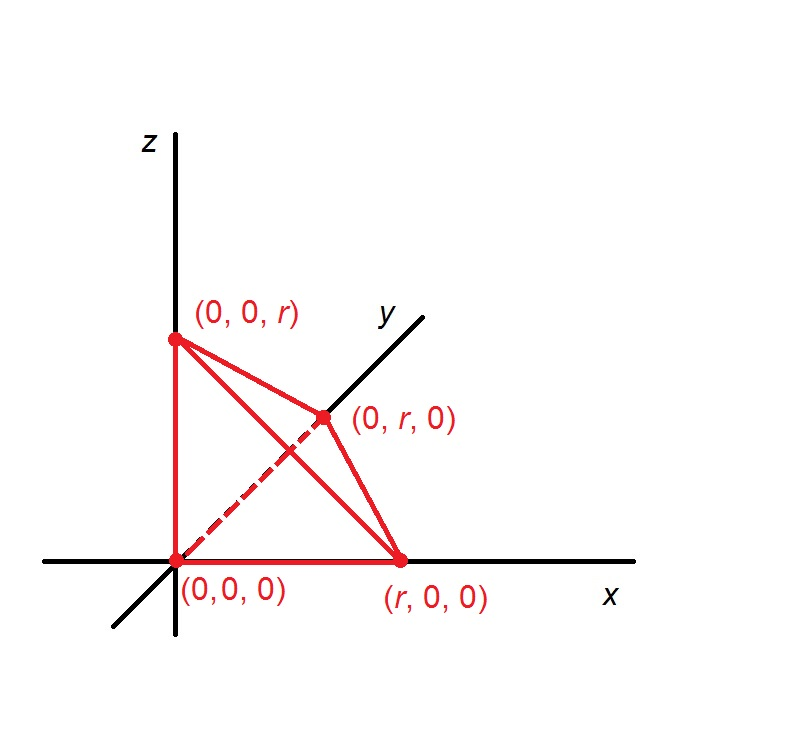The above figure shows a triangular pyramid, or tetrahedron, on the three-dimensional coordinate axes. The tetrahedron has volume 1,000. Which of the following is closest to the value of?Explanation:

If we take the triangle on the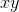-plane to be the base of the pyramid, this base has legs both of length; its area is half the product of the lengths which is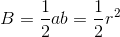Its height is the length of the side along the-axis, which is also of lengthThe volume of a pyramid is equal to one third the product of its height and the area of its base, so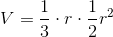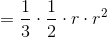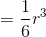Setting the volumeequal to 1,000, we can solve for: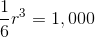Multiply both sides by 6: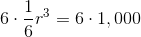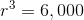Take the cube root of both sides: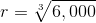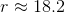The closest choice is 20.

2 Next →